Oct 21, 2021

# Ethereum’s London Hard Fork and the Actual Gains - A Quantitative Deep Dive

After our last look at EIP-1559 we’ve had a chance to read some reactions in the community and were particularly intrigued by Vitalik’s Reddit Post about the 9% increase in chain capacity post EIP-1559.

Since Vitalik’s post estimated ranges for how significantly different factors of the upgrade were impacting the throughput, we thought perhaps we could try to nail down more concretely how much each factor was contributing.

## Methodology

As with our last post, we’ll be sourcing data from the Unbounded Network, but unlike the EIP-1559 post, we’ll be using daily summarized data rather than going block by block to better line up with methodology of Vitalik’s post.

For the purposes of ‘pre-fork’ and ‘post-fork’ analysis, we’ll be excluding the daily summaries for the actual day of the fork, since it contains a mix of both.

## Analysis

So, our first natural question would be, did chain capacity really increase 9%? Given what we looked at last time, it should be pretty simple to confirm. Let’s start by reproducing the Total Gas Usage chart.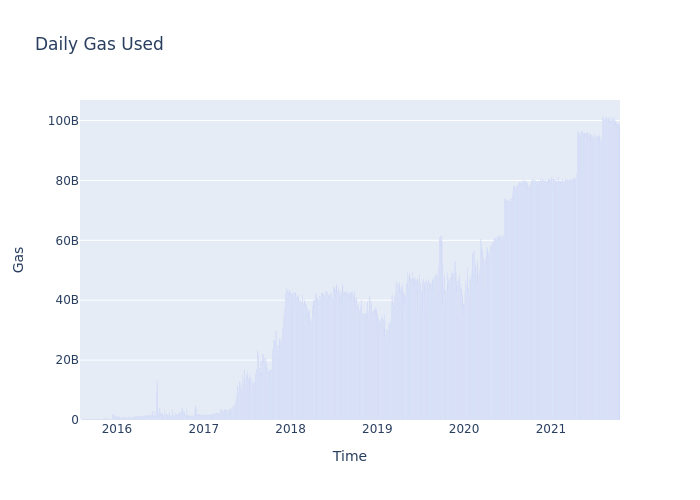We can see the familiar curve of increasing gas usage, followed by plateaus, and hard forks. Let’s go ahead and zoom in a bit around the London Fork to see more precisely what’s changed.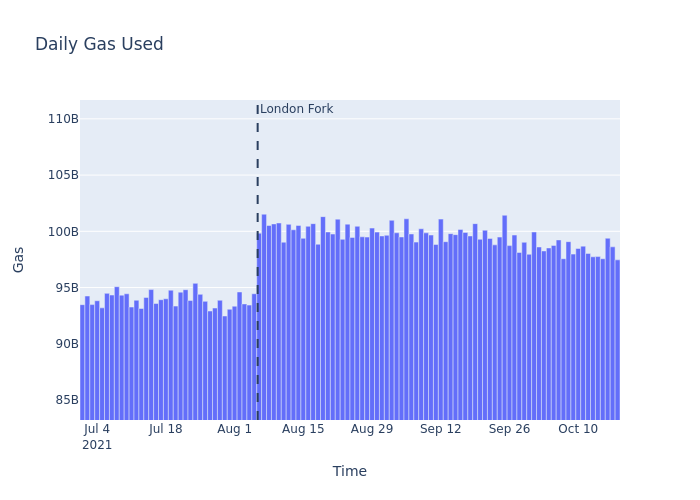As anticipated, we see the gas usage spike from about 93.4 billion the day before the fork to 99.8 billion the day after. Based on the plot, it’s clear that the days leading up to the fork had some of the lowest gas usage in recent history, so it’s probably more fair to compare with a larger sample.

We’ll simply take the mean daily gas usage from July 1st through August 4th, and compare it to the mean from August 6th forward to compute the additional throughput we’re seeing.

```Pre-fork mean gas usage: 93.913540986 billion
Post-fork mean gas usage: 99.53761449412163 billion
Percent change: 6.0%```

Whereas Vitalik’s post claims “~92B to ~100B: a 9% increase”, using more exacting gas figures, we can see the increase in throughput is actually less than 7%.

Of course, even a 7% change in throughput is significant, so let’s try to break down exactly how that change is occuring. In his analysis Vitalik attributes the increase in total gas usage to three components:

1. The Ice Age Delay
2. Target vs. Maximal Blocks
3. Imperfect Math in the Base Fee Adjustment

We’ll see later in the analysis that these three factors are actually comingled a bit, but let’s try to analyze each in isolation first.

### 1. The Ice Age Delay

Wait, what’s ‘the ice age’? For those newer initiants of the Ethereum community, there is something in the Ethereum specification called a ‘Difficulty Bomb’. Although dramatically named, the difficulty bomb is a simple exponential function which is applied to scale the difficulty of the Proof of Work mining. As time progresses, unless defused via a hard fork, the difficulty bomb makes blocks harder to mine and therefore appear further and further apart until effectively, the chain becomes frozen (the Ice Age). This mechanic forces the Ethereum community to periodically hard fork the chain. For any subset of the network which does not fork, mining blocks will rapidly become impractical. Of course, this ‘bomb’ does not prevent a subset of the network from forking to their own rules, but it ensures that a zombie pre-fork network does not simply continue to exist out of apathy rather than intent. The key point is that blocks get produced more slowly over time, a little bit slower at first, but eventually much much slower.

So, blocks are produced more slowly over time until the difficulty bomb resets, let’s plot it!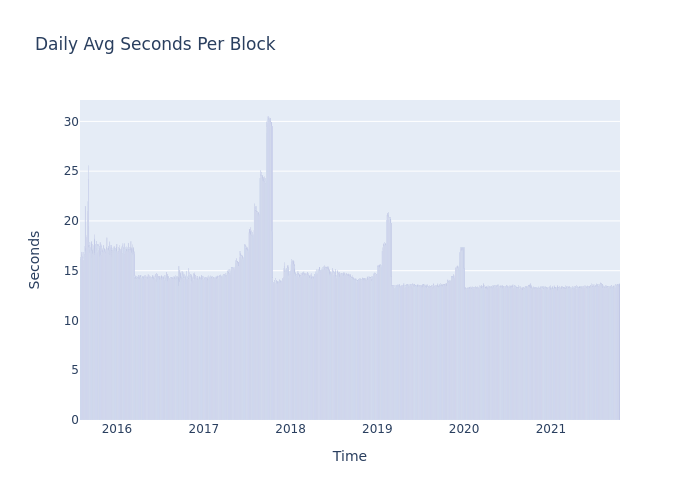Great! But what we’ve actually plotted is the inverse relationship to what we actually care about. We want to know how many blocks are being created per unit of time, not how many units of time per block. So, let’s instead take a look at blocks per day, and zoom in a bit closer to the London Hard Fork to see things a bit more clearly.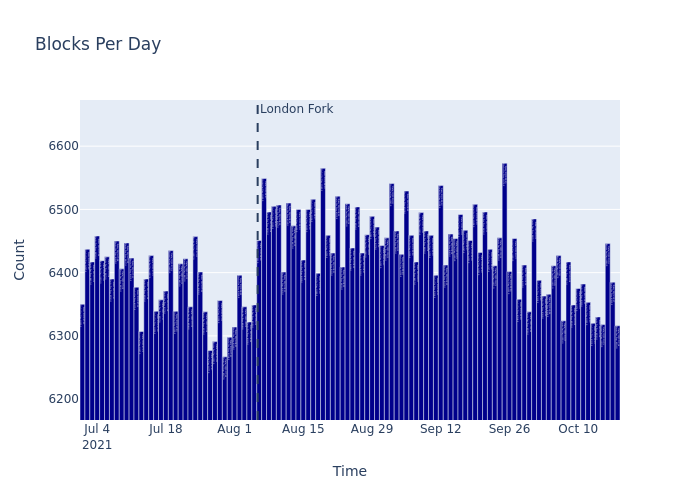In this closer view, it’s clear that post-London, there are in fact more blocks being produced per day, but as before, let’s compute the actual mean from July 1st through August 4th for our pre-fork value, and August 6th on for the post-fork value, then compare the two to find out just how many more blocks.

```Mean blocks per day pre-London-fork: 6377.40
Mean blocks per day post-London-fork: 6442.95
Change: 1.03%```

So, the block creation rate has increased by 1.59% at the time of this writing, which falls a bit short of the 3% from Vitalik’s analysis, but as we’ll see in the next sections because this effect is per unit time (and not per block) its multiplicitive effect is more significant than it might initially seem.

### 2. Target vs. Maximal Blocks

We already looked with a great deal of detail about the actual block distribution pre and post London Fork in our last EIP-1559 analysis, and we saw that indeed, almost all blocks were entirely full pre-fork, but a non-negligible number were empty.

Vitalik’s post referenced an analysis from April about the mining of empty blocks in Ethereum, and postulated that around 2-3% of pre-fork blocks were empty. Let’s take a look at the data!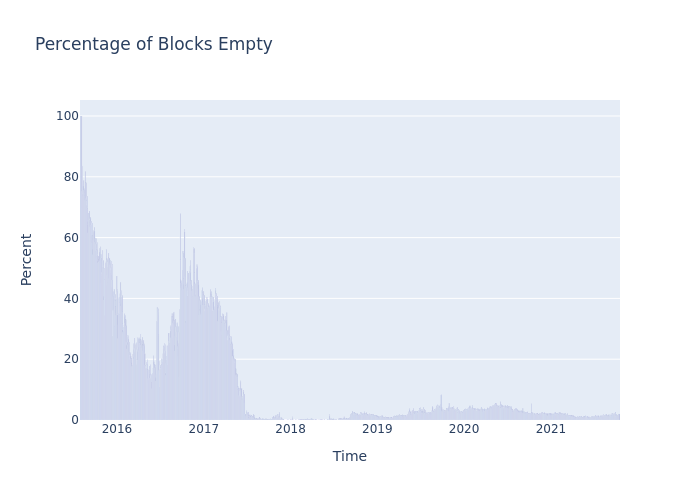We can see that Ethereum has had plenty of empty blocks. But the prevelance of empty blocks appears to have been trending down since late 2020. Let’s zoom in and see how things change around the fork.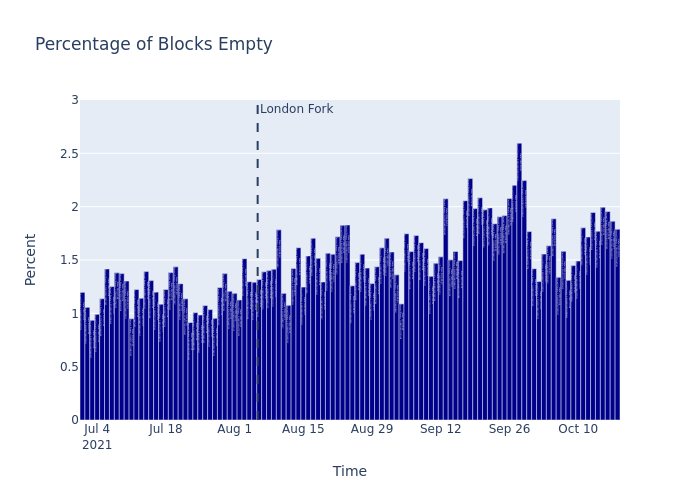We can see that certainly, the pre-fork average does not exceed 1.5% and the fork doesn’t appear to have had a radical impact on the prevelance of empty blocks (though perhaps a slight increaes in frequency). As usual, let’s compute the actual percentage directly by dividing the number of empty blocks by the total number for both pre and post-fork days.

```Percent blocks empty pre-London-fork: 1.19
Percent blocks empty post-London-fork: 1.65
Percent increase if empty blocks were full: 1.21```

As we could intuit from the plot, the percentage of empty blocks pre-fork is actually fairly low, at 1.19%. This increases a bit to 1.43% post-fork (at the time of this writing) but we still fall short of the postulated 2-3% empty blocks.

It’s worth re-iterating that the increase in empty blocks post-fork actually does not impact the total gas throughput, since London introduces the notion of a gas target and any empty blocks actually incentivize the creation of future more full blocks.

Side Note: We’ve computed “Percent increase if empty blocks were full” separately, since for positive values a percentage increase from A to B is always slightly larger than a corresponding percentage decrease from B to A.

So, if empty blocks were as full on average as their non-empty counterparts, we would have seen a 1.21% increase in gas usage, but we were really expecting more. So what are we missing? Let’s consider the theoretical maximum capacity of the prefork chain at 15 million total gas used per block.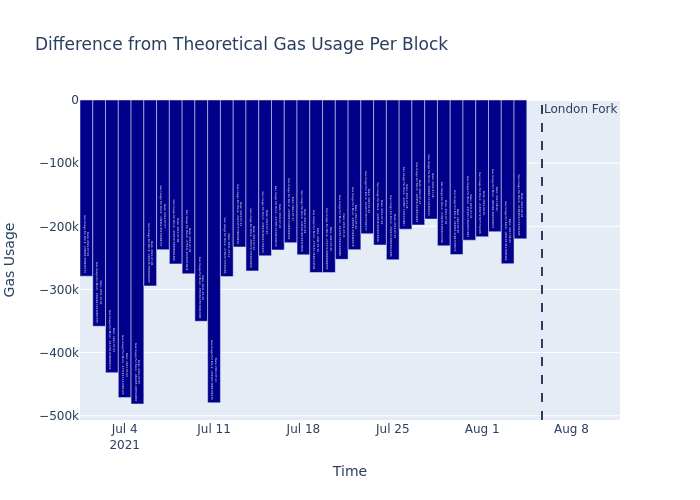Based on this plot we can see that there’s a few hundred thousand units of gas unused each day relative to the maximum theoretical output. Let’s compute exactly what percentage in throughput we would have seen if the pre-London miners had always filled blocks to 100% capacity.

`Percent increase to theoretical pre-fork capacity: 1.86%`

So this gives us a number a bit closer to what we were expecting. If the pre-fork network were performing at the theoretical max gas throughput on every block, then we would expect to see a 1.86% percent increase in throughput. (That’s 0.65% more than can be attributed to empty blocks alone).

### 3. Mathematic Imperfections

Although called out separately from the Max vs. Target gas throughput above, the Mathematical Imperfections are really just the post-fork side of the same coin. While before the London hard fork blocks were always below 15M gas (and therefore the average was below 15M), post-London we want to know how far above/below this same target gas point blocks are actually being mined.

We know:

• The overall increase in throughput (6.7%)
• The increase in block rate (1.6%)
• The potential throughput lost to pre-fork inefficiencies (1.9%)

So, without any more work, we could simply conclude the percentage of post-fork effeciences must satisfy `0.0159*(1+x+0.0186) = 0.067` and solve for `x` to be 3.2%. But that’s less satisfying than computing it explicitly. So instead, let’s see how much the new average block gas usage differs from the theoretical target of 15 million.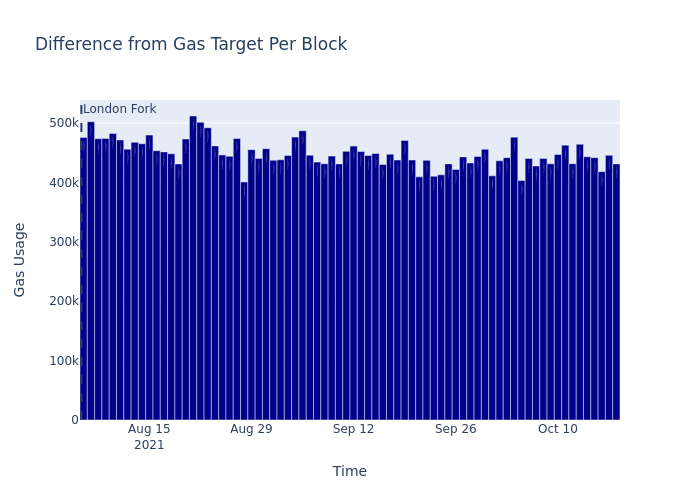Just as expected, the actual average gas used per block exceeds the target gas usage of 15M by several hundred thousand. Computed explicitly:

`Percent increase beyond post-fork target capacity: 2.99%`

For those paying very close attention, you might remember that we predicted 3.2% of the increase to be attributable to the mathematical imperfections, but we see 3.13% above. That is because we’ve computed the additional capacity beyond the theoretical target, not beyond the pre-London usage. If instead we compare it to the mean gas usage per block pre-London, we get:

`Percent increase in post-fork capacity compared to pre-fork capacity: 3.0`

Although that’s what we predicted, we still have one piece left. The extra gas per block is amplified by the additional block rate. So, if we combine it with our previous figures, 1.9% for not filling blocks to max capacity pre-London, and 3.2% for overfilling blocks post-London, we get an overall increase of 5.1% more gas used per block, then multiplied by 1.6% more blocks per day, we finally get to our original 6.7% increase in chain throughput.

```Observed increase in throughput is 5.99%
Computed increase in throughput is 5.99%```

Now finally, let’s have just one more chart, or rather, let’s combine the charts of the missing max theoretical gas throughput pre-fork, and the extra mathematical imperfections post-fork.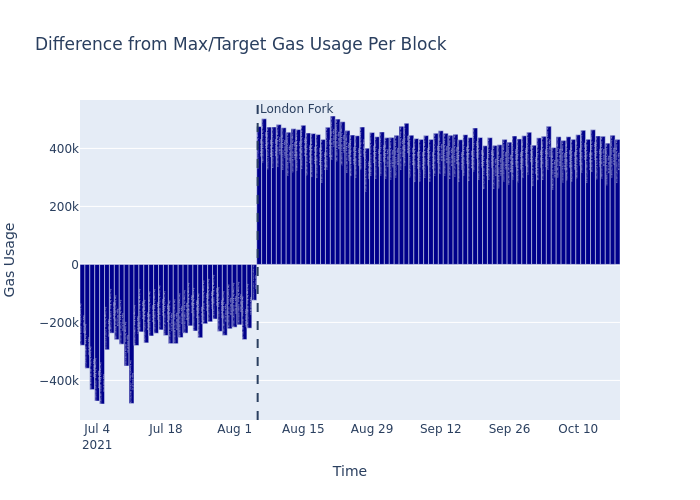This view makes it clear what we stated in the introduction – the difference between max gas and target gas, and the mathematic imperfections are just two sides of the same coin with pre-fork undershooting the target by a bit, while post-fork overshooting. It’s also clear visually that the inefficiencies of the pre-fork max gas limit are significant, but the mathematical imperfections actually contribute a bit more to the overall throughput increase.

## Conclusions

As Vitalik posted, the increase in transaction throughput after the London fork can be split across the three components of Ice Age delay, Max vs. Target, and Mathematical Imperfections. The Mathematical Imperfections are more significant than the Max vs. Target discrepencies, but together they account for the additional gas used per block. Further, we noted that although the Ice Age delay only increases the block production rate by about 1.6%, because it’s a multiplicative factor its effect is magnified by the increased per block gas usage.

## Future Work (Ethereum’s ‘Ice Age’ & the Delay in Merge)

Ethereum’s difficulty bomb refers to the increasing difficulty level or complexity of “puzzles” in the proof-of-work mining algorithm. As the calculations become harder, it results in longer than normal block times and even lower rewards for miners.

We are planning another thorough analysis that we will publish here too. Follow us to get notified when it’s published.